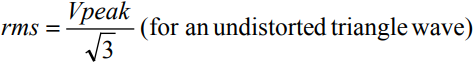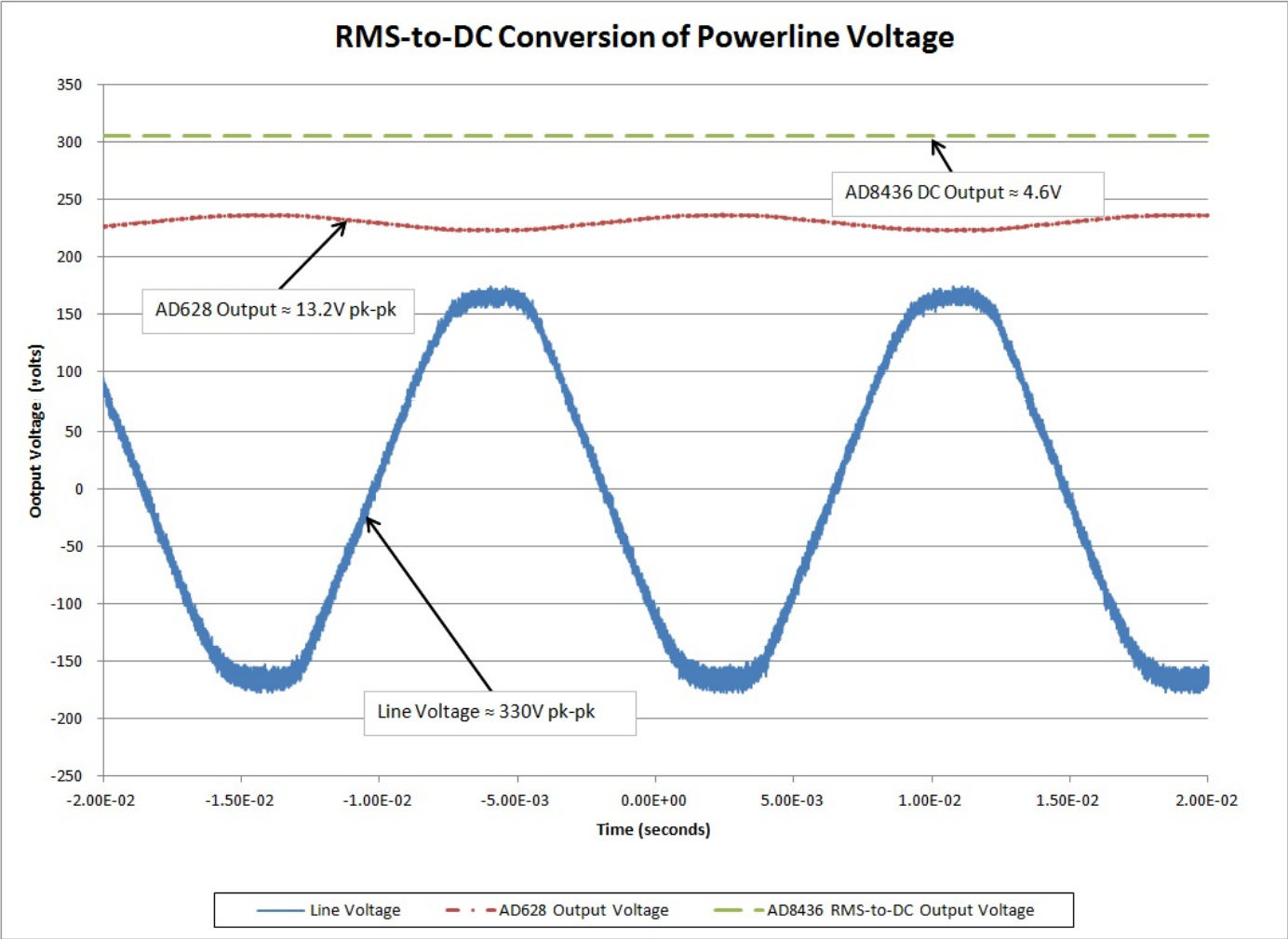Simple Circuit Measures the RMS Value of an AC Power Line

The rms value of an ac signal compares the heating value of an unknown ac signal to that of a known dc signal across identical loads and is equal to the amount of dc required to produce an identical amount of heat in the load. When the power dissipated in the loads is equal, the known dc voltage equals the rms value of the unknown ac signal. For example, if we applied 1 V ac rms to a resistive heating element, it would produce exactly the same amount of heat as if we had applied 1 V dc.

Mathematically, the rms value of a voltage is defined asThis formula represents the standard deviation of a zero-average statistical signal.

Simpler relationships include the following:In general, measuring the rms value requires an rms-to-dc converter, which provides a dc output equal to the rms value of any input waveform. Unfortunately, the range of ac signals to be measured can be very large, while the input range of typical rms-to-dc converters is only a few volts. To be useful for rms-to-dc converters, the large input voltages must, thus, be scaled down. Measuring the rms value of a home power line, for example, requires addtional circuitry that attenuates the ac signal to a suitable value that accomodates the input range of the rms-to-dc converter. This application solves the problem of rms measurements for large ac signals such as those from the electric power line.

In Figure 1, the AD628 programmable-gain difference amplifier, configured for a gain of 1/25, scales the power line signal before applying it to the AD8436 rms-to-dc converter, which can only accept voltages within 0.7 V of either supply. The difference amplifier has a ±120-V common-mode input and differential-mode range, making it well suited for dividing down the high-voltage power line. The precise dc equivalent of the rms value of the ac waveform is provided at RMS OUT. Figure 2 shows the 330-V ac p-p, 60-Hz home power line, the scaled output from the difference amplifier, and the dc output of the rms-to-dc converter.Figure 1. Simple Circuit Measures the RMS Value of a Power Line.Figure 2. Input, Intermediate, and Output Waveforms.

The complete design draws only 2 mA, making it ideal for low-power applications. The external input resistor, 150 kΩ as shown, can be scaled up for use with signals larger than 400 V p-p. The input signal can exceed the power supply with no damage to the device, allowing the input signal to be present even in the absence of the supply voltage. In addition, the short-circuit protected system can operate on dual supplies up to ±18 V.

This circuit computes the true root-mean-square of a complex ac (or ac plus dc) input signal and gives an equivalent dc output level. The true rms value of a waveform is a more useful quantity than the average rectified value because it is a measure of the power in the signal. The rms value of an ac-coupled signal is also its standard deviation.